# Python modules for Statistics¶

## NumPy¶

NumPy is short for Numerical Python, is the foundational package for scientific computing in Python. It contains among other things:

• a powerful N-dimensional array object
• tools for integrating C/C++ and Fortran code
• useful linear algebra, Fourier transform, and random number capabilities

Besides its obvious scientific uses, NumPy can also be used as an efficient multi-dimensional container of generic data. Arbitrary data-types can be defined. This allows NumPy to seamlessly and speedily integrate with a wide variety of databases.

## SciPy¶

SciPy is a collection of packages addressing a number of different standard problem domains in scientific computing. Here is a sampling of the packages included:

• scipy.integrate : numerical integration routines and differential equation solvers.
• scipy.linalg : linear algebra routines and matrix decompositions extending beyond those provided in numpy.linalg.
• scipy.optimize : function optimizers (minimizers) and root finding algorithms.
• scipy.signal : signal processing tools.
• scipy.sparse : sparse matrices and sparse linear system solvers.
• scipy.special : wrapper around SPECFUN, a Fortran library implementing many common mathematical functions, such as the gamma function.
• scipy.stats : standard continuous and discrete probability distributions (density functions, samplers, continuous distribution functions), various statistical tests, and more descriptive statistics.
• scipy.weave : tool for using inline C++ code to accelerate array computations.

• scipy.cluster : Clustering algorithms

• scipy.fftpack : Fast Fourier Transform routines
• scipy.integrate : Integration and ordinary differential equation solvers
• scipy.interpolate : Interpolation and smoothing splines
• scipy.ndimage : N-dimensional image processing optimize Optimization and root-finding routines
• scipy.spatial : Spatial data structures and algorithms

SciPy Reference Guide

## pandas¶

pandas provides rich data structures and functions designed to make working with structured data fast, easy, and expressive. It is, as you will see, one of the critical in-gredients enabling Python to be a powerful and productive data analysis environment. pandas combines the high performance array-computing features of NumPy with the flexible data manipulation capabilities of spreadsheets and relational databases (such as SQL). It provides sophisticated indexing functionality to make it easy to reshape, slice and dice, perform aggregations, and select subsets of data.

pandas consists of the following things

• A set of labeled array data structures, the primary of which are Series and DataFrame
• Index objects enabling both simple axis indexing and multi-level / hierarchical axis indexing
• An integrated group by engine for aggregating and transforming data sets
• Date range generation (date_range) and custom date offsets enabling the implementation of customized frequencies
• Input/Output tools: loading tabular data from flat files (CSV, delimited, Excel 2003), and saving and loading pandas objects from the fast and efficient PyTables/HDF5 format.
• Memory-efficient “sparse” versions of the standard data structures for storing data that is mostly missing or mostly constant (some fixed value)
• Moving window statistics (rolling mean, rolling standard deviation, etc.)
• Static and moving window linear and panel regression

pandas Documentation

## matplotlib¶

matplotlib is the most popular Python library for producing plots and other 2D data visualizations. It was originally created by John D. Hunter (JDH) and is now maintained by a large team of developers. It is well-suited for creating plots suitable for publication. It integrates well with IPython, thus providing a comfortable interactive environment for plotting and exploring data. The plots are also interactive; you can zoom in on a section of the plot and pan around the plot using the toolbar in the plot window.

# Installing Python modules¶

A lot of well-known packages are available in your Linux distribution. If you want to install say e.g. numpy in Python 3, launch a terminal and type in Debian/Ubuntu

    sudo apt-get install python3-numpy

To install packages from PyPI (the Python Package Index), Please consult the Python Packaging User Guide.

# Working with data¶

## Read and write data in Python with stdin and stdout¶

In :
#! /usr/bin/env python3
# line_count.py
import sys
count = 0
data = []
for line in sys.stdin:
count += 1
data.append(line)
print(count) # print goes to sys.stdout
print(data)

0
[]


Then launch a terminal and first make your Python script executable. Then send you testFile to your Python script

chmod +x line_count.py
cat L3-Python-for-Statistical-Modeling.html | ./line_count.py

## Read from and write to files directly¶

You can also explicitly read from and write to files directly in your code. Python makes working with files pretty simple.

• The first step to working with a text file is to obtain a file object using open()

  file_for_reading = open('reading_file.txt', 'r')



'w' is write -- will destroy the file if it already exists!

  file_for_writing = open('writing_file.txt', 'w')



'a' is append -- for adding to the end of the file

  file_for_appending = open('appending_file.txt', 'a')
• The second step is do something with the file.

• Don't forget to close your files when you're done.

  file_for_writing.close()

Note Because it is easy to forget to close your files, you should always use them in a with block, at the end of which they will be closed automatically:

with open(filename,'r') as f:
data = function_that_gets_data_from(f)
In :
#! /usr/bin/env python3
# hash_check.py
import re
starts_with_hash = 0

# look at each line in the file use a regex to see if it starts with '#' if it does, add 1
# to the count.

with open('line_count.py','r') as file:
for line in file:
if re.match("^#",line):
starts_with_hash += 1
print(starts_with_hash)

2


If your file has no headers (which means you probably want each row as a list , and which places the burden on you to know what's in each column), you can use csv.reader() in csv module to iterate over the rows, each of which will be an appropriately split list.

If your file has headers, you can either skip the header row (with an initial call to reader.next()) or get each row as a dict (with the headers as keys) by using csv.DictReader() in module:

symbol date closing_price AAPL 2015-01-23 112.98 AAPL 2015-01-22 112.4 AAPL 2015-01-21 109.55 AAPL 2015-01-20 108.72 AAPL 2015-01-16 105.99 AAPL 2015-01-15 106.82 AAPL 2015-01-14 109.8 AAPL 2015-01-13 110.22 AAPL 2015-01-12 109.25

In :
#! /usr/bin/env python3

import csv

data = {'date':[], 'symbol':[], 'closing_price' : []}
with open('stocks.csv', 'r') as f:
data['date'].append(row["date"])
data['symbol'].append(row["symbol"])
data['closing_price'].append(float(row["closing_price"]))

In :
data.keys()

Out:
dict_keys(['date', 'symbol', 'closing_price'])

Alternatively, pandas provides read_csv() function to read csv files

In :
#! /usr/bin/env python3

import pandas

print(len(data2))
print(type(data2))

16556
<class 'pandas.core.frame.DataFrame'>


The pandas I/O API is a set of top level reader functions accessed like read_csv() that generally return a pandas object. These functions includes

read_excel



See pandas IO tools for detailed explanation.

# Linear Algebra¶

Linear algebra can be done conveniently via scipy.linalg. When SciPy is built using the optimized ATLAS LAPACK and BLAS libraries, it has very fast linear algebra capabilities. If you dig deep enough, all of the raw lapack and blas libraries are available for your use for even more speed. In this section, some easier-to-use interfaces to these routines are described.

All of these linear algebra routines expect an object that can be converted into a 2-dimensional array. The output of these routines is also a two-dimensional array.

## Matrices and n-dimensional array¶

In :
import numpy as np
from scipy import linalg
A = np.array([[1,2],[3,4]])
A

Out:
array([[1, 2],
[3, 4]])
In :
linalg.inv(A) # inverse of a matrix

Out:
array([[-2. ,  1. ],
[ 1.5, -0.5]])
In :
b = np.array([[5,6]]) #2D array
b

Out:
array([[5, 6]])
In :
b.T

Out:
array([,
])
In :
A*b #not matrix multiplication!

Out:
array([[ 5, 12],
[15, 24]])
In :
A.dot(b.T) #matrix multiplication

Out:
array([,
])
In :
b = np.array([5,6]) #1D array
b

Out:
array([5, 6])
In :
b.T  #not matrix transpose!

Out:
array([5, 6])
In :
A.dot(b)  #does not matter for multiplication

Out:
array([17, 39])

## Solving linear system¶

In :
import numpy as np
from scipy import linalg
A = np.array([[1,2],[3,4]])
A

Out:
array([[1, 2],
[3, 4]])
In :
b = np.array([,])
b

Out:
array([,
])
In :
linalg.inv(A).dot(b) #slow

Out:
array([[-4. ],
[ 4.5]])
In :
A.dot(linalg.inv(A).dot(b))-b #check

Out:
array([[0.],
[0.]])
In :
np.linalg.solve(A,b) #fast

Out:
array([[-4. ],
[ 4.5]])
In :
A.dot(np.linalg.solve(A,b))-b #check

Out:
array([[0.],
[0.]])

## Determinant¶

In :
import numpy as np
from scipy import linalg
A = np.array([[1,2],[3,4]])
linalg.det(A)

Out:
-2.0

## Least-squares problems and pseudo-inverses¶

In :
import numpy as np
from scipy import linalg
import matplotlib.pyplot as plt

In :
c1, c2 = 5.0, 2.0
i = np.r_[1:11]
xi = 0.1*i
yi = c1*np.exp(-xi) + c2*xi
zi = yi + 0.05 * np.max(yi) * np.random.randn(len(yi))

In :
A = np.c_[np.exp(-xi)[:, np.newaxis], xi[:, np.newaxis]]
c, resid, rank, sigma =linalg.lstsq(A, zi)

In :
xi2 = np.r_[0.1:1.0:100j]
yi2 = c*np.exp(-xi2) + c*xi2

In :
plt.plot(xi,zi,'x',xi2,yi2)
plt.axis([0,1.1,3.0,5.5])
plt.xlabel('$x_i$')
plt.title('Data fitting with linalg.lstsq')
plt.show()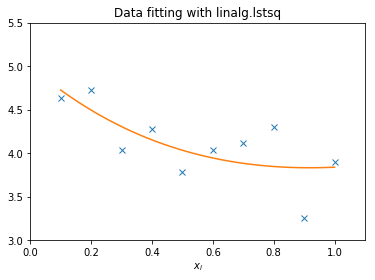## Eigenvalues and eigenvectors¶

In :
import numpy as np
from scipy import linalg
A = np.array([[1,2],[3,4]])
la,v = linalg.eig(A)
l1,l2 = la
print(l1, l2)  #eigenvalues

print(v[:,0])  #first eigenvector

print(v[:,1])  #second eigenvector

print(np.sum(abs(v**2),axis=0)) #eigenvectors are unitary

v1 = np.array(v[:,0]).T
print(linalg.norm(A.dot(v1)-l1*v1)) #check the computation

(-0.3722813232690143+0j) (5.372281323269014+0j)
[-0.82456484  0.56576746]
[-0.41597356 -0.90937671]
[1. 1.]
5.551115123125783e-17


## Singular Value Decomposition (SVD)¶

In :
import numpy as np
from scipy import linalg
A = np.array([[1,2,3],[4,5,6]])

In :
M,N = A.shape
U,s,Vh = linalg.svd(A)
Sig = linalg.diagsvd(s,M,N)

In :
U, Vh = U, Vh
U

Out:
array([[-0.3863177 , -0.92236578],
[-0.92236578,  0.3863177 ]])
In :
Sig

Out:
array([[9.508032  , 0.        , 0.        ],
[0.        , 0.77286964, 0.        ]])
In :
Vh

Out:
array([[-0.42866713, -0.56630692, -0.7039467 ],
[ 0.80596391,  0.11238241, -0.58119908],
[ 0.40824829, -0.81649658,  0.40824829]])
In :
U.dot(Sig.dot(Vh)) #check computation

Out:
array([[1., 2., 3.],
[4., 5., 6.]])

## QR decomposition¶

The command for QR decomposition is linalg.qr.

# Statistical Distributions¶

A large number of probability distributions as well as a growing library of statistical functions are available in scipy.stats. See http://docs.scipy.org/doc/scipy/reference/stats.html for a complete list.

Generate random numbers from normal distribution:

In :
from scipy.stats import norm
r = norm.rvs(loc=0, scale=1, size=1000)


Calculate a few first moments:

In :
mean, var, skew, kurt = norm.stats(moments='mvsk')


Display the probability density function (pdf)

In :
import numpy as np
import matplotlib.pyplot as plt
x = np.linspace(norm.ppf(0.01), #ppf stands for percentiles.
norm.ppf(0.99), 100)

fig, ax = plt.subplots(1, 1)
ax.plot(x, norm.pdf(x),
'blue', lw=5, alpha=0.6, label='norm pdf')
plt.show()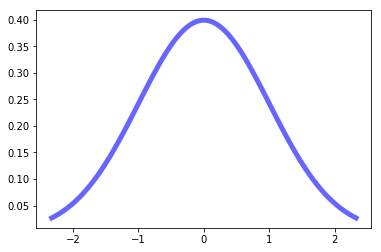And compare the histogram:

In :
fig, ax = plt.subplots(1, 1)
ax.hist(r, density=True, histtype='stepfilled', alpha=1, label='...')
ax.legend(loc='best', frameon=False)
plt.show()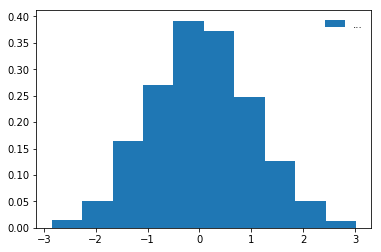# Linear regression model¶

This example computes a least-squares regression for two sets of measurements.

In :
from scipy import stats
import numpy as np
x = np.random.random(10)
y = np.random.random(10)
slope, intercept, r_value, p_value, std_err = stats.linregress(x,y)
print({'slope':slope,'intercept':intercept})
print({'p_value':p_value,'r-squared':round(r_value**2,2)})

{'slope': 0.39840035794159673, 'intercept': 0.23831754280320663}
{'p_value': 0.35978414666977093, 'r-squared': 0.11}


## Optimization¶

The minimize function provides a common interface to unconstrained and constrained minimization algorithms for multivariate scalar functions in scipy.optimize

In :
import numpy as np
from scipy.optimize import minimize

## Define the function
def rosen(x):
"""The Rosenbrock function"""
return sum(100.0*(x[1:]-x[:-1]**2.0)**2.0 + (1-x[:-1])**2.0)

x0 = np.array([1.3, 0.7, 0.8, 1.9, 1.2])

## Calling the minimize() function
options={'xtol': 1e-8, 'disp': True})
print(res.x)

Optimization terminated successfully.
Current function value: 0.000000
Iterations: 339
Function evaluations: 571
[1. 1. 1. 1. 1.]


# Data Visualizing¶

In :
from matplotlib import pyplot as plt
years = [1950, 1960, 1970, 1980, 1990, 2000, 2010]
gdp = [300.2, 543.3, 1075.9, 2862.5, 5979.6, 10289.7, 14958.3]
# create a line chart, years on x-axis, gdp on y-axis
fig = plt.figure()
plt.plot(years, gdp, color='green', marker='o', linestyle='solid')
plt.title("Nominal GDP")
# add a label to the y-axis
plt.ylabel("Billions of \$")
plt.show()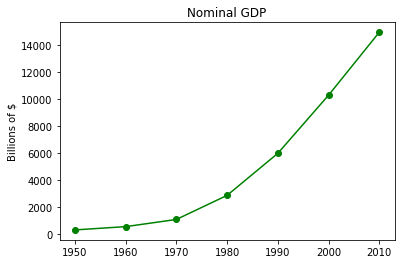## 3D Plot¶

In :
import numpy as np
def f(x, y):
return np.sin(np.sqrt(x ** 2 + y ** 2))

x = np.linspace(-6, 6, 30)
y = np.linspace(-6, 6, 30)

X, Y = np.meshgrid(x, y)
Z = f(X, Y)

In :
import matplotlib.pyplot as plt
from mpl_toolkits.mplot3d import Axes3D
fig = plt.figure()
ax = plt.axes(projection='3d')
ax.plot_surface(X, Y, Z, rstride=1, cstride=1,
cmap='viridis', edgecolor='none')
ax.set_title('surface')

Out:
Text(0.5,0.92,'surface')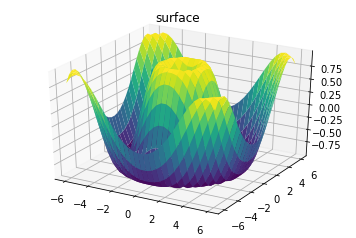In [ ]: